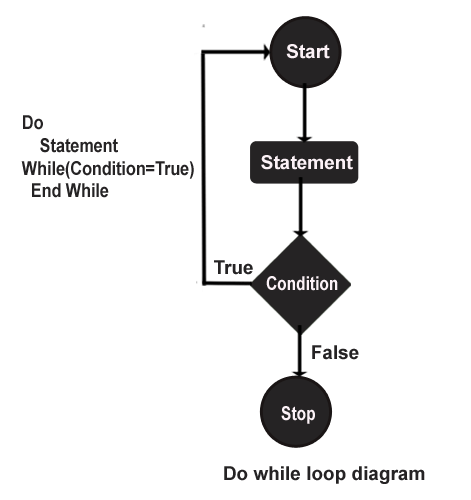Tutorials

# Do-while loop in Java

In this section you will learn how to use the do-while statement in java.

In this section you will learn how to use the do-while statement in java.

## Do-while loop in Java

In this section you will learn about do-while loop in Java. do-while loop is similar to while loop but the difference is do-while loop execute the condition at least once. Some time we need to execute certain condition repeatedly and run at least once. For that we need a do-while loop so that at least once a block of statement will executed. do-while loop execute once without testing the condition but in while loop what happen if the condition is true then only the statement inside the while loop will be executed, otherwise the control will jump outside the while loop.

Syntax:

```do{
statement;
}
while(boolean-expression);```

The boolean expression will execute once before testing the boolean expression, and after that if the boolean expression is true then control will jump up and execute the statement inside the do, until the boolean expression will become false.

do-while loop consist of condition and statement. First the code inside the loop is executed  then the condition is evaluated and if the boolean expression or condition is true then again the code will executed. It will execute until the boolean expression become false. Because do-while loop check the condition after the statement is executed. So while is called pre-test loop and do-while loop is also known as post-test loop. The while loop is a entry-control loop which test the condition first then block inside the loop is executed and do-while loop is a exit-control loop. It means the code always executed first and then the boolean-expression is evaluated. Here is the flow diagram of do while loop as follows:Example : Using this example you will understand the do-while loop easily.

``` public class DowhileExample
{
public static void main(String args[])
{
int i=0;
do
{
System.out.println("Value of i ="+i);
i++;
}while(i<=10);
}
}
```

Output from the program: